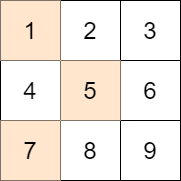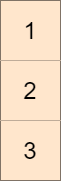Formatted question description: https://leetcode.ca/all/1981.html

# 1981. Minimize the Difference Between Target and Chosen Elements

Medium

## Description

You are given an m x n integer matrix mat and an integer target.

Choose one integer from each row in the matrix such that the absolute difference between target and the sum of the chosen elements is minimized.

Return the minimum absolute difference.

The absolute difference between two numbers a and b is the absolute value of a - b.

Example 1:Input: mat = [[1,2,3],[4,5,6],[7,8,9]], target = 13

Output: 0

*Explanation:8 One possible choice is to:

• Choose 1 from the first row.
• Choose 5 from the second row.
• Choose 7 from the third row.

The sum of the chosen elements is 13, which equals the target, so the absolute difference is 0.

Example 2:Input: mat = [,,], target = 100

Output: 94

Explanation: The best possible choice is to:

• Choose 1 from the first row.
• Choose 2 from the second row.
• Choose 3 from the third row.

The sum of the chosen elements is 6, and the absolute difference is 94.

Example 3:Input: mat = [[1,2,9,8,7]], target = 6

Output: 1

Explanation: The best choice is to choose 7 from the first row.

The absolute difference is 1.

Constraints:

• m == mat.length
• n == mat[i].length
• 1 <= m, n <= 70
• 1 <= mat[i][j] <= 70
• 1 <= target <= 800

## Solution

Use dynamic programming. Create a 2D array dp with m + 1 rows and 4901 columns, where dp[i][j] represents whether sum j is reachable by using the first i rows of mat. Loop over mat to fill in the values in dp. Finally, loop over the last row of dp and find the value j such that dp[m][j] = true and j is closest to target. Return the absolute difference between j and target.

class Solution {
public int minimizeTheDifference(int[][] mat, int target) {
int rows = mat.length, columns = mat.length;
boolean[][] dp = new boolean[rows + 1];
dp = true;
for (int i = 0; i < rows; i++) {
for (int j = 0; j <= 4900; j++) {
if (dp[i][j]) {
for (int k = 0; k < columns; k++)
dp[i + 1][j + mat[i][k]] = true;
}
}
}
int minDifference = Integer.MAX_VALUE;
for (int j = 0; j <= 4900; j++) {
if (dp[rows][j]) {
int difference = Math.abs(j - target);
minDifference = Math.min(minDifference, difference);
}
}
return minDifference;
}
}